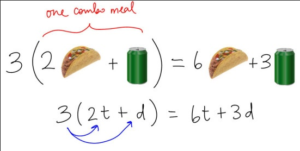## Use the Distributive Property to Simplify

### Learning Outcomes

• Simplify expressions with fraction bars, brackets, and parentheses
• Use the distributive property to simplify expressions with grouping symbols
• Simplify expressions containing absolute values

In this section, we will use the skills from the last section to simplify mathematical expressions that contain many grouping symbols and many operations. We will also use the distributive property to break up multiplication into addition.  Additionally, you will see how to handle absolute value terms when you simplify expressions.

### Example

Simplify $\dfrac{5-[3+(2\cdot (-6))]}{{{3}^{2}}+2}$

The video that follows contains an example similar to the written one above. Note how the numerator and denominator of the fraction are simplified separately.

## The Distributive Property

Parentheses are used to group, or combine expressions and terms in mathematics.  You may see them used when you are working with formulas, and when you are translating a real situation into a mathematical problem so you can find a quantitative solution.Combo Meal Distributive Property

For example, you are on your way to hang out with your friends, and call them to ask if they want something from your favorite drive-through.  Three people want the same combo meal of $2$ tacos and one drink.  You can use the distributive property to find out how many total tacos and how many total drinks you should take to them.

$\begin{array}{c}\,\,\,3\left(2\text{ tacos }+ 1 \text{ drink}\right)\\=3\cdot{2}\text{ tacos }+3\text{ drinks }\\\,\,=6\text{ tacos }+3\text{ drinks }\end{array}$

The distributive property allows us to explicitly describe a total that is a result of a group of groups. In the case of the combo meals, we have three groups of ( two tacos plus one drink). The following definition describes how to use the distributive property in general terms.

### The Distributive Property of Multiplication

For real numbers $a,b$, and $c$:

$a(b+c)=ab+ac$.

What this means is that when a number is multiplied by an expression inside parentheses, you can distribute the multiplier to each term of the expression individually.

To simplify  $3\left(3+y\right)-y+9$, it may help to see the expression translated into words:

multiply three by (the sum of three and $y$), then subtract $y$, then add $9$

To multiply three by the sum of three and $y$, you use the distributive property –

$\begin{array}{c}\,\,\,\,\,\,\,\,\,3\left(3+y\right)-y+9\\\,\,\,\,\,\,\,\,\,=\underbrace{3\cdot{3}}+\underbrace{3\cdot{y}}-y+9\\=9+3y-y+9\end{array}$

Now you can combine the like-terms. Subtract $y$ from $3y$ and add $9$ to $9$.

$\begin{array}{c}\color{blue}{9}\color{red}{+3y-y}\color{blue}{+9}\\=18+2y\end{array}$

The next example shows how to use the distributive property when one of the terms involved is negative.

### Example

Simplify $a+2\left(5-b\right)+3\left(a+4\right)$

## Absolute Value

Absolute value expressions are another method of grouping that you may see. Recall that the absolute value of a quantity is always positive or $0$.

When you see an absolute value expression included within a larger expression, treat the absolute value like a grouping symbol and evaluate the expression within the absolute value sign first. Then take the absolute value of that expression. The example below shows how this is done.

### Example

Simplify $\dfrac{3+\left|2-6\right|}{2\left|3\cdot1.5\right|-\left(-3\right)}$

The following video uses the order of operations to simplify an expression in fraction form that contains absolute value terms. Note how the absolute values are treated like parentheses and brackets when using the order of operations.